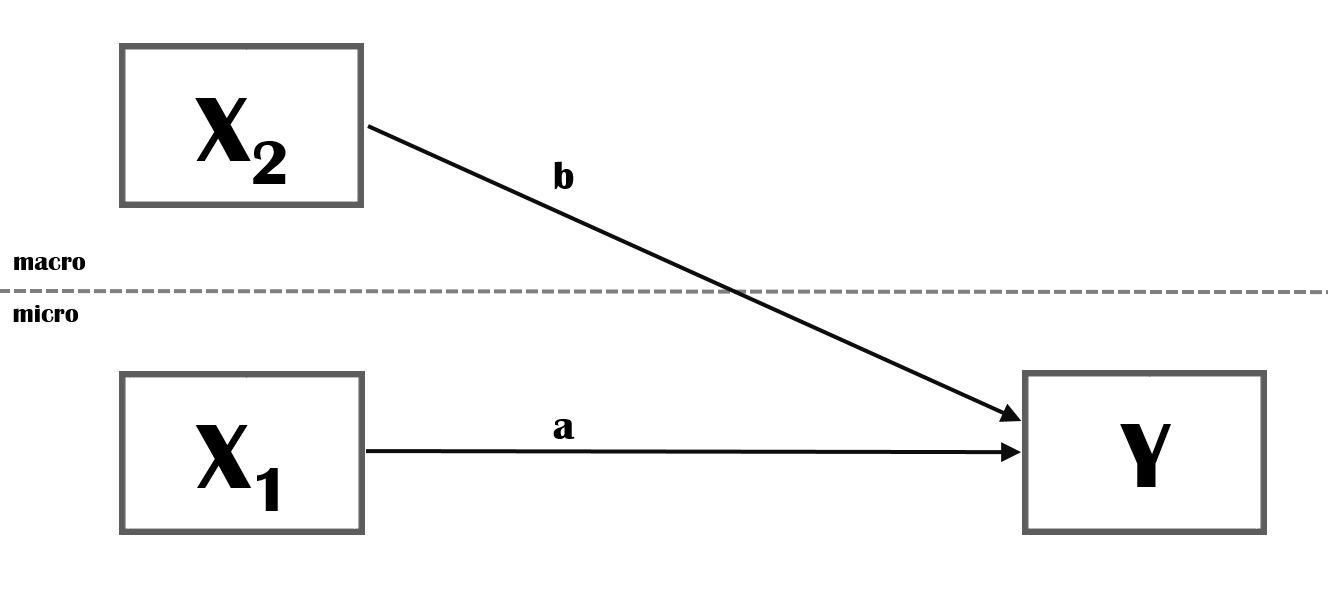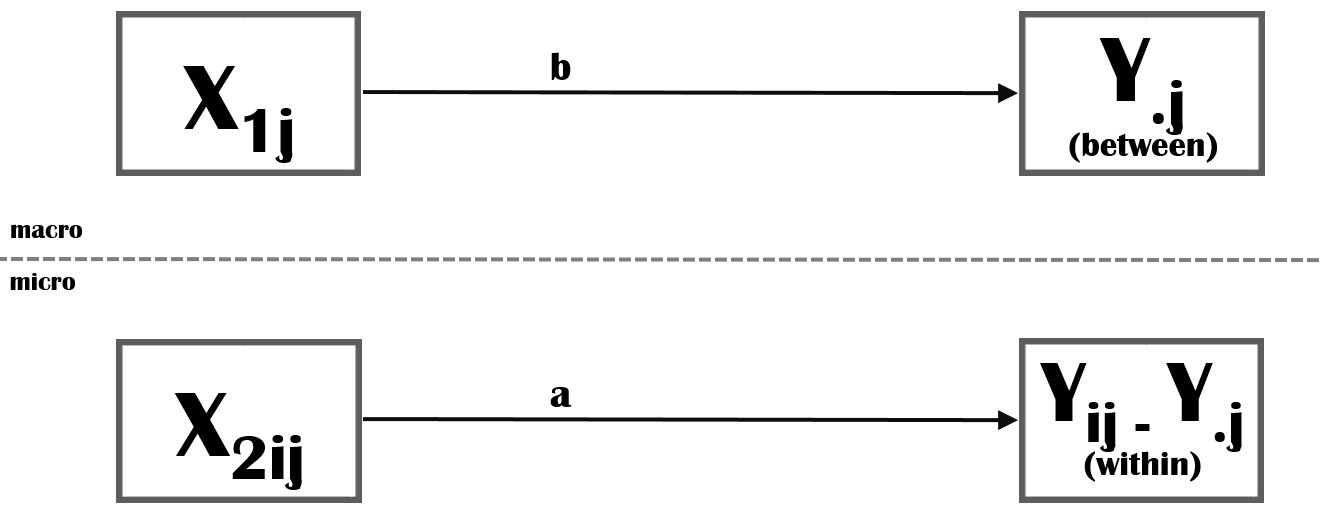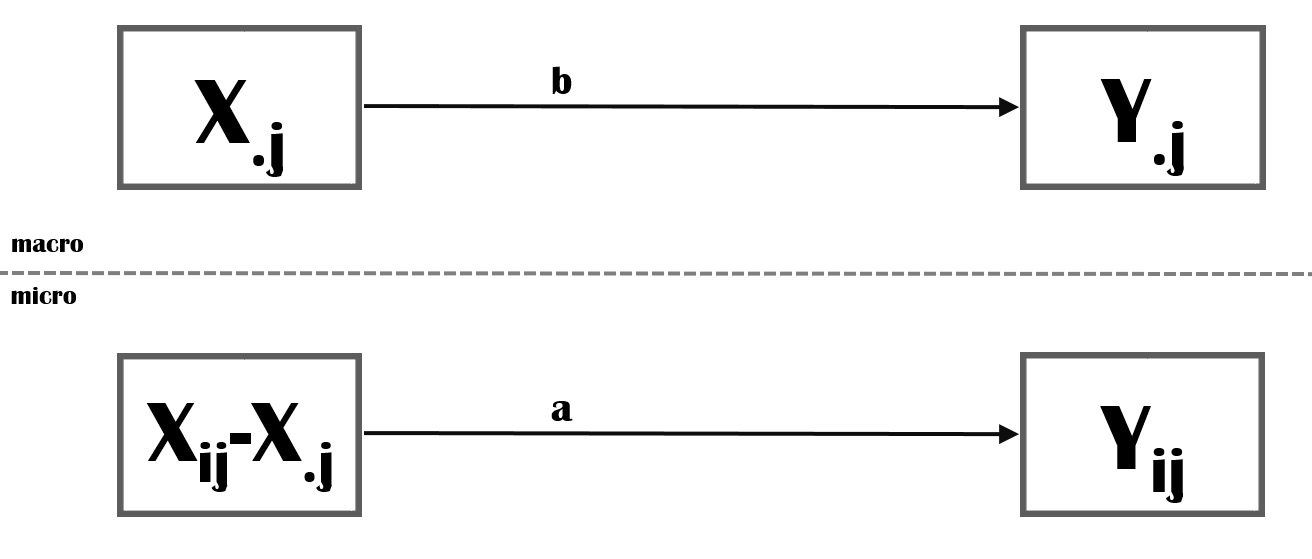# Multilevel

## The Conceptual Model

### Version 1Figure 1: Multi-level model

The independent variable $$X_2$$ is measured at the macro-level (e.g. neighborhood) and impacts the dependent variable, Y, measured at the micro-level (e.g. neighborhood residents). The independent variable $$X_1$$ is measured at the micro-level and impacts the dependent variable, Y, as well.

### Version 2 (preferred)Figure 2: Multi-level model (version 2)

Please note that the independent variable at the macro-level $$X_2$$ will impact all individuals within the same macro-level unit equally. Hence, it impacts the mean score of Y within a macro-level unit. To make it more concrete, a country-level variable like National Income may impact the mean health level of individuals within a country. National Income explains between-country variance in health, not within-country variance in health. To make this more explicit, I recommend you to use the conceptual model above.

### Version 3 (subscripts)Figure 3: Multi-level model (version 3)

Unfortunately, readers who look at version 2 may now think you have two different dependent variables, one at the micro-level and one at the macro-level. You could add subscripts to make more explicit what you mean.
In the figure above we make explicit that we have a dependent variable, Y, that is measured at the micro-level. We explain (between-level) variance in mean scores of Y at the macro-level. The subscript j indexes the different macro-level units. $$Y_{.j}$$ is shorthand notation for mean score of $$Y_{ij}$$ for unit j.
At the micro-level we explain (within-level) variance in deviation-scores from the macro-level unit mean.
The subscripts for the independent variables make clear we have two independent variables, one measured at the macro-level and one measures at the micro-level.

In my experience, readers who do not immediately grasp version 2 don’t understand version 3 either. I would therefore recommend sticking to version 2 and perhaps add between and within in the boxes. Add a note to your conceptual model and explain in words what you mean with your labels.

### Independent micro-level variableFigure 4: Multi-level model (version 3)

So far, we only made explicit that our dependent variable (measured at the micro-level) can be split in a within and between component. But the same holds true for independent variables measured at the micro-level.

Independent variables measured at the micro-level may impact:

1. within macro-unit level variation in the dependent variable
2. between macro-unit level variation in the dependent variable

Ad1: The between macro-level unit variation in the IV may be related to the between macro-level unit variation in the DV. Stated otherwise, the mean score of our IV across the macro-level units may be related to the mean scores of our DV across the macro-level units. This is path b in the figure above.
Ad2: The within macro-level unit variation in the IV may be related to the within macro-level unit variation in the DV. This is path a in the figure above.

Normally, path b is forgotten. Drawing a conceptual model may help you to remember to at least consider this possible pathway. If you do not include this relationship you are actually implicitly assuming that path a and b are the same. If this is not the case your estimation of path a will be biased.

Composition effect: It could be possible that path b is not significant but that between-unit variation in your dependent variable is still explained after inclusion of your independent variable measured at the micro-level. This is then because of a so-called composition effect: the macro-level units harbor different micro-level units (e.g. some neighborhoods harbor more high income residents than others.)

Random samples: To estimate unbiased estimates it is important that you have excess to a random sample of macro-level units (not only high-income neighborhoods) and random samples of micro-level units within each macro-level unit. If the latter is not the case, for example when you sampled relatively rich residents of poor neighborhoods and relatively poor residents of rich neighborhoods, the (estimated or constructed) variable $$X_{.j}$$ will not be a valid measure for the true mean value of all $$X_{ij}$$ in neighborhood j.

work in progress